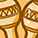cancel
Showing results for
Search instead for
Did you mean:New Contributor

## Potentiometry Half Reaction and Ksp

I am a BS Chemistry student and recently we are studying potentiometry. Since half-reactions are reversible, do we always use the reduction reaction in writing half-reactions, or do we base the reaction on whether they oxidized or reduced? For example, the reaction of quinhydrone is Q + 2H^2+ + 2e- <--> QH, where Q= quinone and HQ= hydroquinone. When writing equations, how will I know that I should rather write the reverse? I am asking this because Ksp=[product]/[reactant], but my textbook says that for this, Ksp= [aQ][aH^2+]^2 / [aQH], so I am wondering how that happened.